Area Of A Square - Definition with Examples

The Complete K-5 Math Learning Program Built for Your Child

• 30 Million Kids

Loved by kids and parent worldwide

• 50,000 Schools

Trusted by teachers across schools

• Comprehensive Curriculum

Aligned to Common Core

What is Area of a Square?

Area of a two-dimensional shape is the space occupied by it. In the given square, the space shaded in blue is the area of the square.The space occupied by the swimming pool below can be found by finding the area of the pool.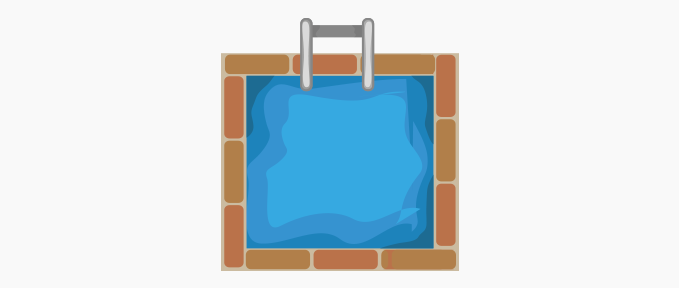Or we can calculate the area of a square field to find the number of saplings to be planted. We measure the area in square units

By finding the number of unit squares that are required to fill a shape, we can find its area. The total number of unit squares in a shape give its area.

Consider a square of each side 3 cm long. It can be filled with 3 rows and 3 columns of unit squares.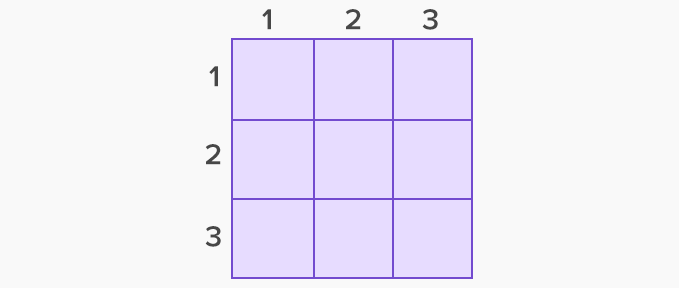The square can be shown on top of a 10 x 10 grid with shaded inside the square.

Area of each unit square = 1 sq. cm

No. of squares = 3 x 3 = 9

So, total area = 9 sq. cm

In other words, the area of a square is the product of the length of each side with itself. That is,  Area A = s x s where s is the length of each side of the square.

For example, the area of a square of each side of length 8 feet is 8 times 8 or 64 square feet.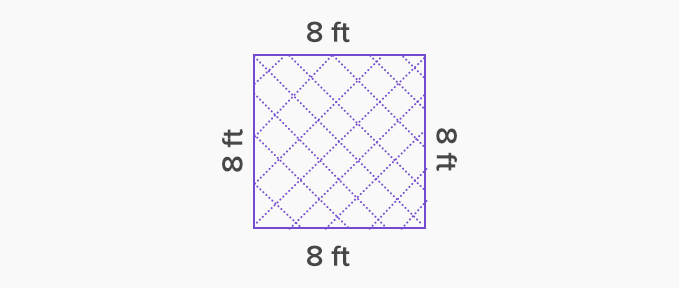Let us solve another example. A square shaped chocolate cake in needs chocolate icing on top. If each side of the cake is 18 inches long, what is the area of the cake that needs icing?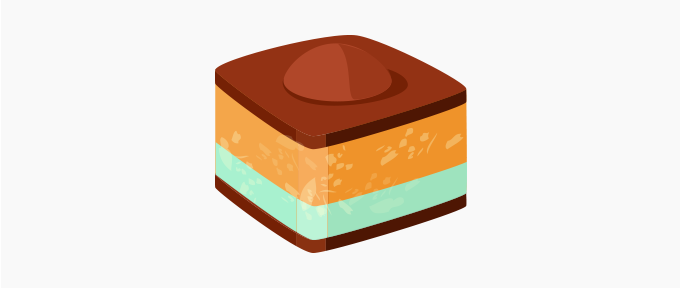A simple square chocolate cake, preferably the vertical sides covered with colorful gems/kitkat

The area covered in chocolate icing is equal to the area of the square on top of the cake.

Length of each side = 18 in.

Area = 18 x 18 = 324 sq. in.

Therefore, the top area of the cake that will need icing will be 324 square inches.

 Fun Facts Each diagonal divides a square into two congruent isosceles right triangles. Each of these right triangles has base and height both equal to the length of each side of the square. So, if the square has each side s units long, then the area of each triangle equals 1⁄2 x s x s =  1⁄2 s2. Since the two triangles are congruent, they have equal area. Thus, the total area of the square is 1⁄2 s2 +  1⁄2 s2 = s2 .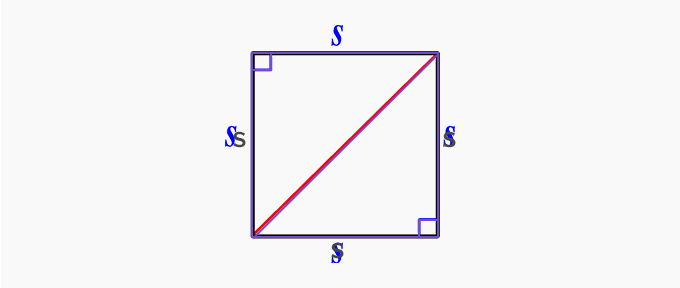Won Numerous Awards & Honors